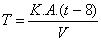### Diary## An Elementary Consideration of Heat Losses from Streams of Water in Caves

Our next article is an example of the scientific approach to caving problems. Members of the B.E.C. are paying increasing attention to this side of caving, and this article is both appropriate and topical

by Mike Luckwill.

Conditions of heat exchange in a large cave are extremely complex and will not be unravelled until a large number of temperature measurements, together with water flow rates and other variables, have been obtained.  The construction of an extremely simple model enables hypotheses to be tested and may point the direction in which results are required. I therefore make no apologies for the crudity of the model I shall investigate.  I will, however, attempt to examine the consequences of the over simplification involved.

First of all, let us look at some facts.  The temperature differences concerned are so small that heat losses due to radiation are negligible and so we need only consider heat losses due to conduction. Water conducts heat about twice as well as rock and about twenty times as well as air.  We shall assume that the water loses heat only to the rock and that the rock is capable of absorbing this heat without increasing in temperature. This assumption is quite reasonable when one considers the vast quantities of rock in relation to the small amount of heat. Furthermore, we shall assume that the temperature of the rock remains constant at 8°C.

We shall assume that the greater mass of water has a temperature t°C and that this temperature only starts to drop within 1 cm of the water/rock surface, giving a temperature gradient of (t-8) °C per cm.

The temperature drop of the water is then given by T where°C/sec. where K = Coefficient of conductivity of water = 0.0015.

A = Water/Rock surface area and V = volume of water.

Let us consider a semicircular channel, radius r cm and length l cm.

The A = prl and V = pr21/2 hence A/V = 2/r.

T therefore = 0.0015(t-8)2/rOC/second and, if r = 40cms, T = 0.000075(t-8)OC/second.

The correct method of finding the manner in which the temperature varies with time would be to use the calculus.  However, in the interests of simplicity, I will make an approximation by assuming that the water stays at the same temperature for ten minutes, and calculate the temperature drop every ten minutes.  Thus,

T = 0.000075(t-8).600OC/10 minutes or 0.045(t-8)OC/10min.

Graph 1 expresses the above resultWe now construct a graph showing the change of temperature of the water with time by the following method.    A time O, we shall assume that the water temperature is 12OC.    From graph 1, we find that the temperature drop at 12OC is 0.18OC.  Subtracting 0.18 from 12 we get 10.86, and we plot this as the temperature for time 10 minutes.  We now find the temperature drop for 10.86 and calculate the temperature at 20 minutes, and so on.  By this method, we obtain graph 2Let us now consider water percolating through rock.  Typical dimensions for a suitable model would be a cylinder of water radius 1cm.  Once again, A = 2prl and V = pr2l, giving

A/V = 1/r = 1 in this case.

Hence T= 0.0015(t-8)OC/second = 0.9(t-8)OC/10 minutes.

Thus, at 12OC, a drop of 3.6OC might occur in ten minutes.  Using the same methods as we did to produce graph 2, we can now produce graph 3, which has been plotted on the same graph above, again showing the change in temperature with time.

What conclusions are to be drawn from these results?  Firstly, a stream arising from percolating water will quickly reach the rock temperature. Thus, the temperature of the rock may be measured by measuring the temperature of a suitable water inlet in the cave e.g. the drinking fountain in St. Cuthberts.  Secondly, the temperature of such a stream is likely to be 1OC lower than the temperature of the Main Stream in the cave, as the main stream would take several hours to reach rock temperature.

To construct a simple model to find air temperature is more difficult.  I suggest that deep in a cave, the air reaches the rock temperature of approximately 8OC.  Accepted temperatures of about 11OC are probably wrong because of the difficulty of measurement.  As soon as one approaches a thermometer near enough to read it, ones breath must quickly cause the thermometer to give a false reading.

## Book Review

### DOOLIN  ST. CATHERINES CAVE.  by Dr. O.C. Lloyd, published by the U.B.S.S. at ten shillings.

This is yet another very worth while publication by the U.B.S.S. on one of the caves of County Claire.  The publication consists of thirty pages of text, together with eight photographic plates and a grade 4 survey.

The bulk of the text is devoted to a detailed description of the cave system and an explanation of its origin.  This is preceded by a history of the exploration of the system in which is included a song inspired by the Doolin Cave and a description of the cave rescue carried in 1957.

The cave consists of nearly five miles of passages and any person proposing to explore the system would be well advised to make reference to this publication.